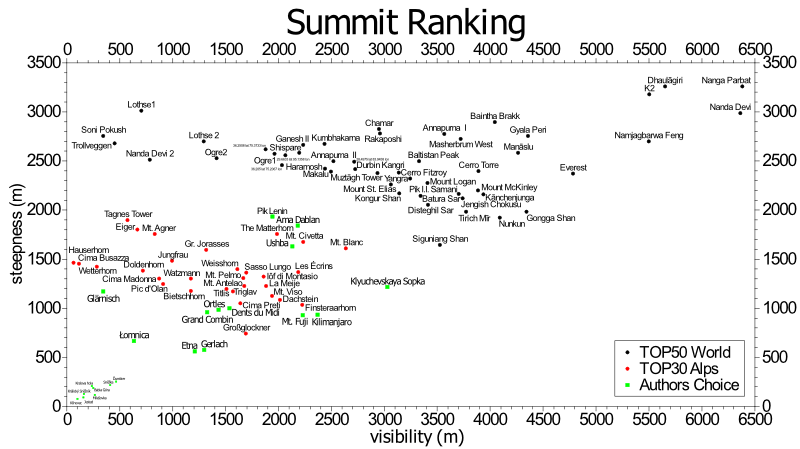﻿ $$\def\bold#1{\bf #1} \newcommand{\d}{\mathrm{d}}$$ World summit ranking

#### Power Uphill

bike mass [kg]
body mass [kg]
altitude gain [m]
climb length [km]
time [s]
speed [km/h]
power [W]
power/mass [W/kg]
climbrate [m/min]

### World summit ranking

This is about the most amazing peak on earth. There might be no surprise at the top summits, but maybe you find some interesting things you didn't know before.Based on the extraordinary work of http://www.viewfinderpanoramas.org it was possible to analyse the whole globe and categorize every single hill. The calculation on the Intel i7 took around 20 hours, but result proves for example wikipedias claim, that the Nanga Parbat is the worlds larges mass elevation.

$steep~wall = \sqrt{\left\langle \left(\max_r\left(\frac{\Delta h(r,\varphi)^2}{r}\right)\right)^2\right\rangle_\varphi}$ $visibility = \int\int{dA \left(\frac{\Delta h(\vec{r})}{r}\right)^2\frac{1}{2\pi r}}$ $pyramide = \left\langle \max_r\left(\frac{\Delta h(r,\varphi)^2}{r}\right)\right\rangle_\varphi-\left\langle \left(\max_r\left(\frac{\Delta h(r,\varphi)^2}{r}\right)-\left\langle \max_r\left(\frac{\Delta h(r,\varphi)^2}{r}\right)\right\rangle_\varphi\right)^2\right\rangle_\varphi$ $total = \sqrt{steepwall^2+pyramide^2+visibility^2}$

The formulas are applied upon the base area of the mountain, e.g. at all locations $$\vec{r}=(r,\varphi)$$ which directly guide by gradient to the peak or which guide to a child peak, but lie below its prominence level.